# as a varies from 0 to 8.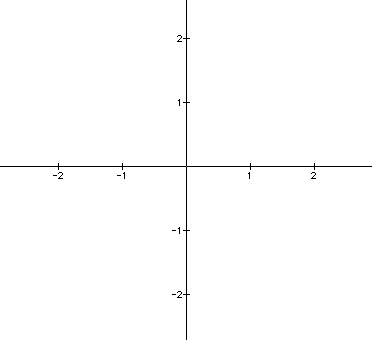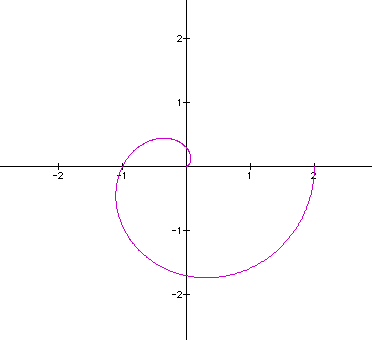a = 0 a = 0.5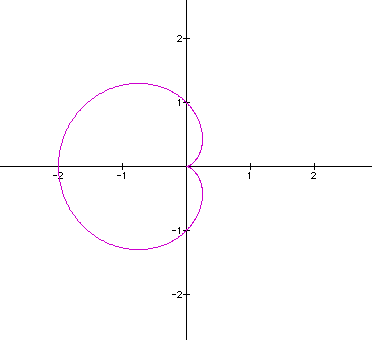a = 1.0 a = 1.5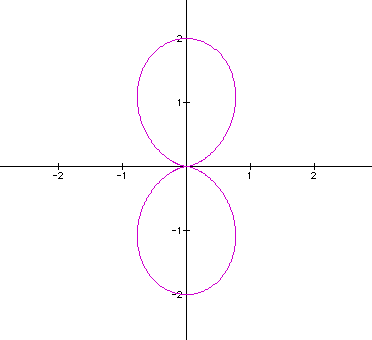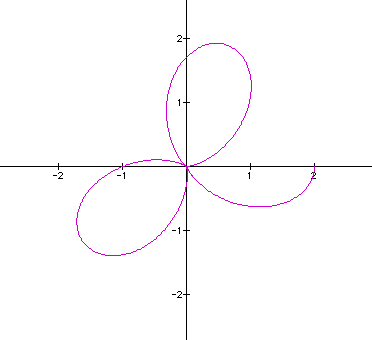a = 2.0 a = 2.5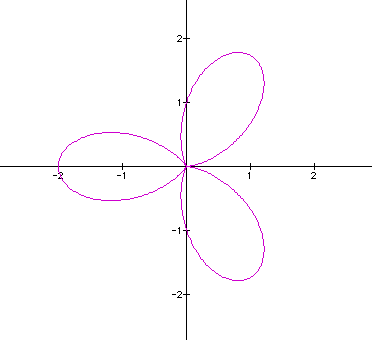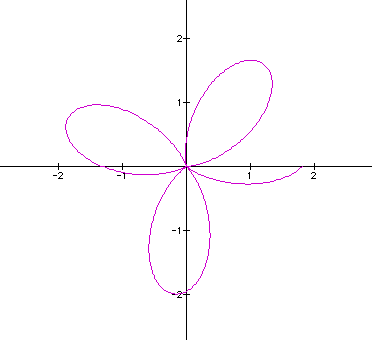a = 3.0 a = 3.5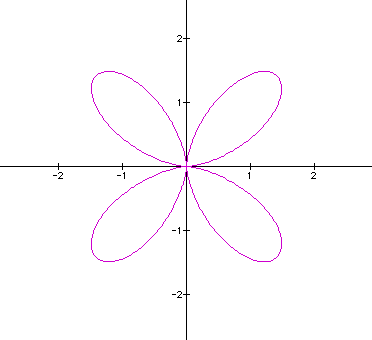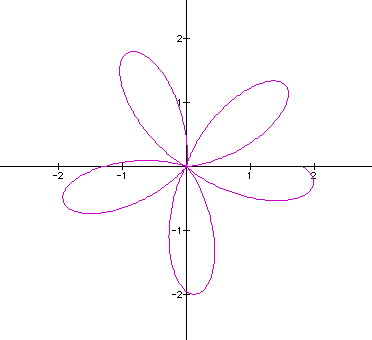a = 4.0 a = 4.5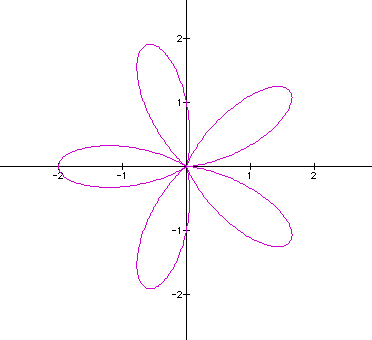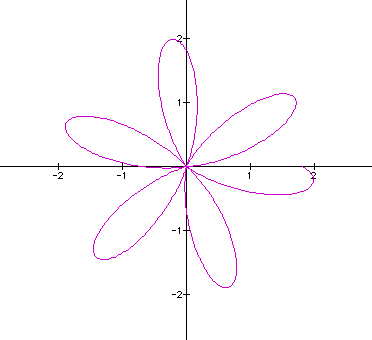a = 5.0 a = 5.5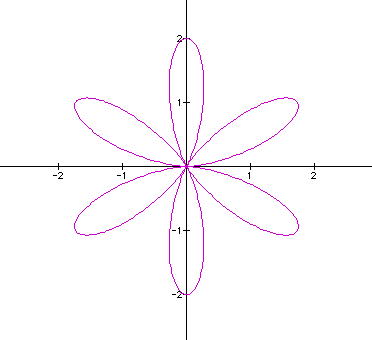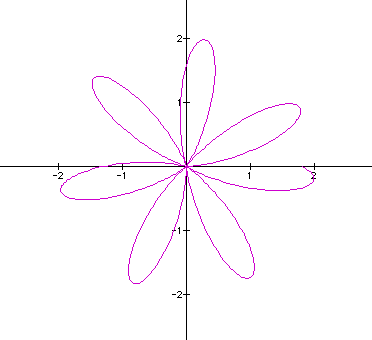a = 6.0 a = 6.5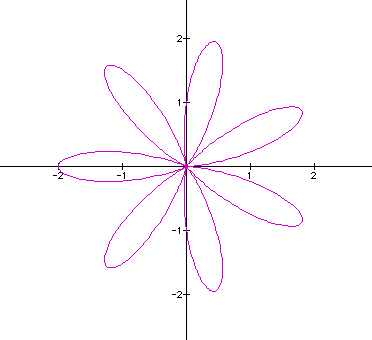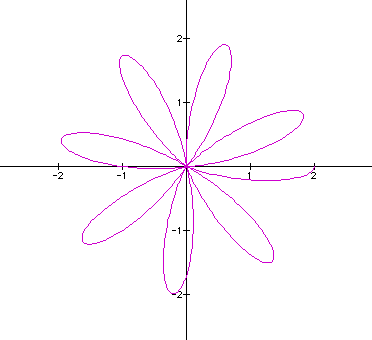a = 7.0 a = 7.5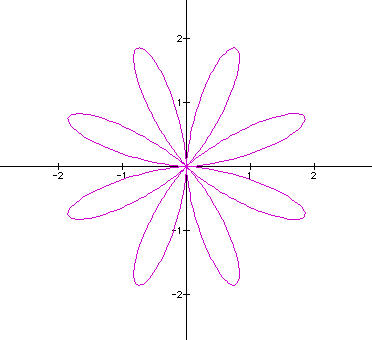a = 8.0

See a QuickTime movie of what happens to the cardiod as a varies from 0 to 10.